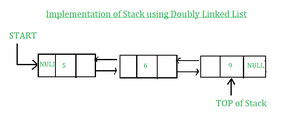# Python | Stack using Doubly Linked List

A stack is a collection of objects that are inserted and removed using Last in First out Principle(LIFO). User can insert elements into the stack, and can only access or remove the recently inserted object on top of the stack. The main advantage of using LinkedList over array for implementing stack is the dynamic allocation of data whereas in the array, the size of the stack is restricted and there is a chance of stack overflow error when the size of the stack is exceeded the maximum size.

### Stack Operations:

1. push() : Insert the element into Stack and assign the top pointer to the element.
2. pop() : Return top element from the Stack and move the top pointer to the second element of the Stack.
3. top() : Return the top element.
4. size() : Return the Size of the Stack.
5. isEmpty() : Return True if Stack is Empty else return False.
6. printstack() : Print all elements of the stack.

### Below is the implementation of the above-mentioned stack operations using Doubly LinkedList in Python:Implementation of Stack using Doubly Linked List

## Python3

 `# A complete working Python program to demonstrate all ` `# stack operations using a doubly linked list `   `# Node class ` `class` `Node:`   `# Function to initialise the node object` `    ``def` `__init__(``self``, data):` `        ``self``.data ``=` `data ``# Assign data` `        ``self``.``next` `=` `None` `# Initialize next as null` `        ``self``.prev ``=` `None` `# Initialize prev as null        ` `        `  `# Stack class contains a Node object` `class` `Stack:` `    ``# Function to initialize head ` `    ``def` `__init__(``self``):` `        ``self``.head ``=` `None` `        `  `# Function to add an element data in the stack ` `    ``def` `push(``self``, data):`   `        ``if` `self``.head ``is` `None``:` `            ``self``.head ``=` `Node(data)` `        ``else``:` `            ``new_node ``=` `Node(data)` `            ``self``.head.prev ``=` `new_node` `            ``new_node.``next` `=` `self``.head` `            ``new_node.prev ``=` `None` `            ``self``.head ``=` `new_node` `            `  `            `  `# Function to pop top element and return the element from the stack ` `    ``def` `pop(``self``):`   `        ``if` `self``.head ``is` `None``:` `            ``return` `None` `        ``elif` `self``.head.``next` `is` `None``:` `            ``temp ``=` `self``.head.data` `            ``self``.head ``=` `None` `            ``return` `temp` `        ``else``:` `            ``temp ``=` `self``.head.data` `            ``self``.head ``=` `self``.head.``next` `            ``self``.head.prev ``=` `None` `            ``return` `temp`         `# Function to return top element in the stack ` `    ``def` `top(``self``):`   `        ``return` `self``.head.data`     `# Function to return the size of the stack ` `    ``def` `size(``self``):`   `        ``temp ``=` `self``.head` `        ``count ``=` `0` `        ``while` `temp ``is` `not` `None``:` `            ``count ``=` `count ``+` `1` `            ``temp ``=` `temp.``next` `        ``return` `count` `    `  `    `  `# Function to check if the stack is empty or not  ` `    ``def` `isEmpty(``self``):`   `        ``if` `self``.head ``is` `None``:` `           ``return` `True` `        ``else``:` `           ``return` `False` `            `    `# Function to print the stack` `    ``def` `printstack(``self``):` `        `  `        ``print``("stack elements are:")` `        ``temp ``=` `self``.head` `        ``while` `temp ``is` `not` `None``:` `            ``print``(temp.data, end ``=``"``-``>")` `            ``temp ``=` `temp.``next`           `        `    `# Code execution starts here         ` `if` `__name__``=``=``'__main__'``: `   `# Start with the empty stack` `  ``stack ``=` `Stack()`   `# Insert 4 at the beginning. So stack becomes 4->None ` `  ``print``("Stack operations using Doubly LinkedList")` `  ``stack.push(``4``)`   `# Insert 5 at the beginning. So stack becomes 4->5->None ` `  ``stack.push(``5``)`   `# Insert 6 at the beginning. So stack becomes 4->5->6->None ` `  ``stack.push(``6``)`   `# Insert 7 at the beginning. So stack becomes 4->5->6->7->None ` `  ``stack.push(``7``)`   `# Print the stack` `  ``stack.printstack()`   `# Print the top element` `  ``print``("\nTop element ``is` `", stack.top())`   `# Print the stack size` `  ``print``("Size of the stack ``is` `", stack.size())`   `# pop the top element` `  ``stack.pop()`   `# pop the top element` `  ``stack.pop()` `  `  `# two elements are popped` `# Print the stack` `  ``stack.printstack()` `  `  `# Print True if the stack is empty else False` `  ``print``("\nstack ``is` `empty:", stack.isEmpty())`   `#This code is added by Suparna Raut`

Output:

```Stack operations using Doubly LinkedList
stack elements are:
7->6->5->4->
Top element is  7
Size of the stack is  4
stack elements are:
5->4->
stack is empty: False```

Time Complexity for operations:

• Push(): O(1)
• pop(): O(1)
• top(): O(1)
• size(): O(N)
• isEmpty(): O(1)
• printStack(): O(N)

Auxiliary Space for operations:

• Push(): O(1)
• pop(): O(1)
• top(): O(1)
• size(): O(1)
• isEmpty(): O(1)
• printStack(): O(1)

Feeling lost in the world of random DSA topics, wasting time without progress? It's time for a change! Join our DSA course, where we'll guide you on an exciting journey to master DSA efficiently and on schedule.
Ready to dive in? Explore our Free Demo Content and join our DSA course, trusted by over 100,000 geeks!